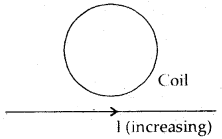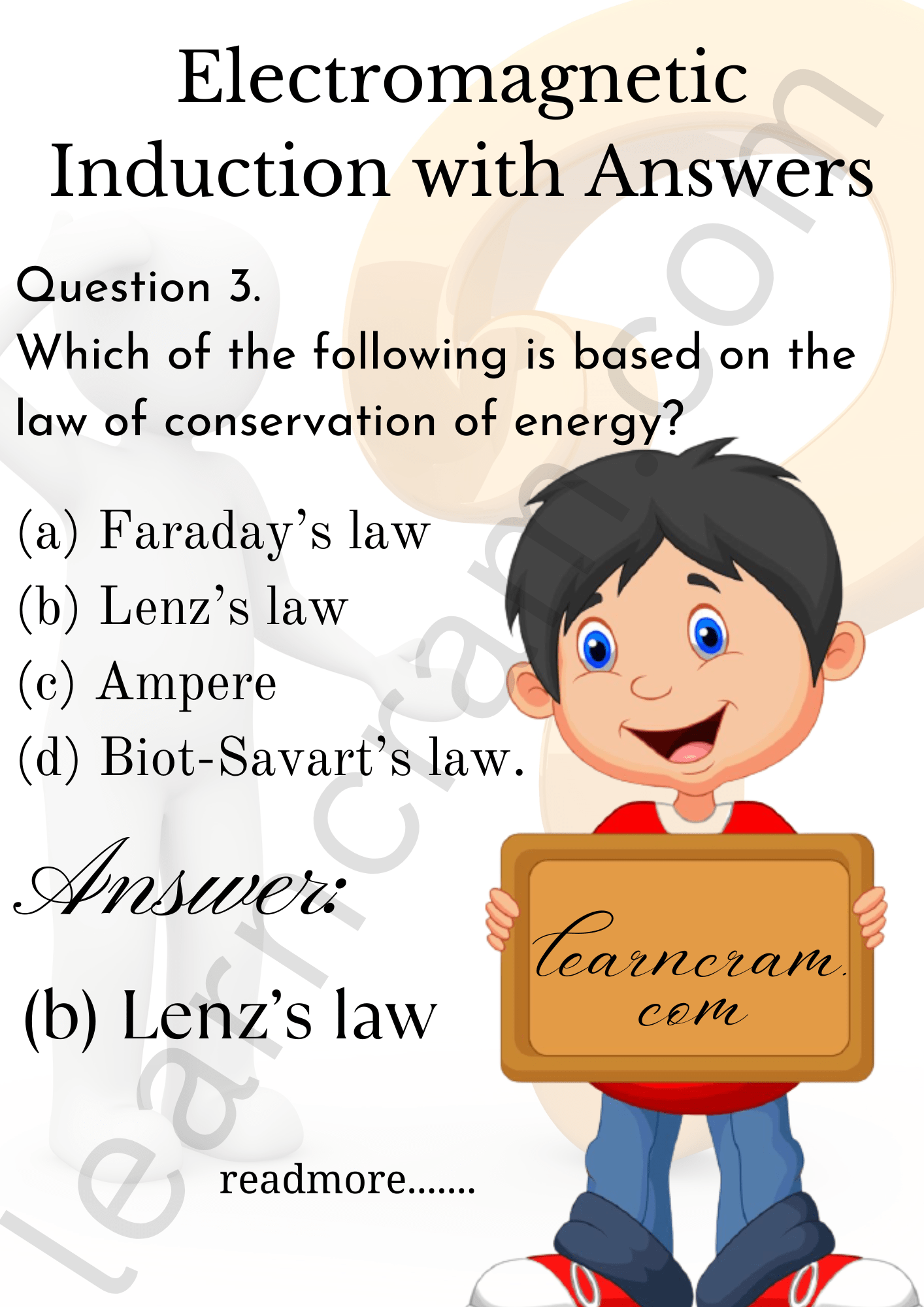# MCQ Questions for Class 12 Physics Chapter 6 Electromagnetic Induction with Answers

We have compiled the NCERT MCQ Questions for Class 12 Physics Chapter 6 Electromagnetic Induction with Answers Pdf free download covering the entire syllabus. Practice MCQ Questions for Class 12 Physics with Answers on a daily basis and score well in exams. Refer to the Electromagnetic Induction Class 12 MCQs Questions with Answers here along with a detailed explanation.

## Electromagnetic Induction Class 12 MCQs Questions with Answers

Question 1.
A circular coil is placed near a straight conductor as shown below. When the current in the straight conductor increases, the current in the coil is(a) clockwise
(b) anticlockwise
(c) normal to the plane oi coil
(d) None of them.Question 2.
Which of the following gives the direction of the induced e.m.f.?
(b) Lenz’s law
(c) Ampere
(d) Biot-Savart’s law.Question 3.
Which of the following is based on the law of conservation of energy?
(b) Lenz’s law
(c) Ampere
(d) Biot-Savart’s law.

Question 4.
The electric current in a circuit varies from + 2A to – 2A in a time 10-2 s. Another coil of resistance 20 Ω and inductance 2H is placed hear it. What will be the induced current in the second coil?
(a) 4A
(b) 8A
(c) 20A
(d) 40A

Question 5.
Which of the following instrument do not make use of eddy currents?
(a) Electrical brakes
(c) inductor motor
(d) Transformer.

Question 6.
The motional e.m.f. is the induced e.m.f.
(a) in a circuit due to variation in its own current.
(b) in a circuit due to variation of current in the neighbouring circuit.
(c) in a coil due to the motion of the magnet near it.
(d) across the ends of a wire moving in a magnetic field.

Answer: (d) across the ends of a wire moving in a magnetic field.

Question 7.
Whaf voltage is developed across the axle of the wheels of a train, when it moves with a speed of 72 kmh-1? The horizontal component of earth’s magnetic field is 0.40 × 10-4 T and the angle of dip is 30°. The length of the axle is 1.5 m.
(a) 0.8 mv
(b) 0.4 mv
(c) 0.2 mv
(d) 0.7 mv

Question 8.
Eddy currents produced in a conductor are responsible for:
(a) damping
(b) loss of energy
(c) heating
(d) All of the above

Answer: (d) All of the above

Question 9.
A car moves up on a plane road. The induced e.m.f. in the axle connecting the two wheels is maximum, when it moves:
(a) At the poles
(b) At equator
(c) remains stationary
(d) No e.m.f. is induced at all.

Question 10.
When the current through a solenoid increases at a constant rate, the induced current:
(a) is a constant and is in the direction of inducing current.
(b) is a constant and is opposite to the direction of the inducing current.
(c) increases with time and is in the direction of the inducing current.
(d) increases with time and is opposite to the direction of the inducing current.

Answer: (b) is a constant and is opposite to the direction of the inducing current.

Question 11.
Which of the following is/are equal to Henry?
(a) Volt second/ampere.
(b Volt(second)²/coulomb.
(c) Joule (second)²/(coulomb)²
(d) All of these

Question 12.
A coil of metal wire is kept stationary in a non-uniform magnetic field:
(a) An e.m.f. and current both are induced in the coil.
(b) An e.m.f. but not the current is induced iii the coil.
(c) Current but not the e.m.f is induced in the coil.
(d) Neither e.m.f. nor current is induced in the coil.

Answer: (d) Neither e.m.f. nor current is induced in the coil.

Question 13.
Two different coils have self-inductances L1 = 8 mH, L2 = 2 mH. The current in one coil is increased at a constant rate. The current in the second coil is also increased at the same constant rate. At a certain instant of time, the power given to the two coils is the same. At that time, the current, the induced voltage and energy stored in the two coils are I1, V1, U1, and I2, V2, U2 respeactively.
(a) $$\frac {V_2}{V_1}$$ = $$\frac {1}{4}$$
(b) $$\frac {I_1}{I_2}$$ = $$\frac {1}{4}$$
(c) $$\frac {U_2}{U_1}$$ = 4
(d) All of the Above

Answer: (d) All of the Above

Question 14.
Induced e.m.f. produced in a coil rotating in a magnetic field will be maximum when the angle between the axis of coil and direction of magnetic field is:
(a) 90°
(b) 45°
(c) 0°
(d) 180°

Question 15.
A copper rod moves parallel to the horizontal direction. The induced e.m.f. developed across its ends due to earth’s magnetic field will be maximum at the:
(a) poles
(b) equator
(c) latitude 30°
(d) latitude 60°.

Question 16.
A coil having 500 square loops each of side 10 cm is placed normal to the magnetic field which increases at a rate of 1.0 Wb s-1. The induced e.m.f. is;
(a) 0.1V
(b) 5 V
(c) 0.5 V
(d) 1.0 V

Question 17.
When current I is passed through an inductor of coefficient of self-inductance L, energy stored in it is $$\frac {1}{2}$$ Lt². This energy stored
is in the form of:
(a) Voltage
(b) Current
(c) Magnetic field
(d) Electric field.

Fill in the Blanks

Question 1.
If a core of soft iron is introduced into a coil its coefficient of self induction gets ……………….

Question 2.
Two circular conductors A and B are placed perpendicular to each other as shown in the figure. If the current in one of the coils is changed, then current induced in the other coil is equal to ……………….Question 3.
The coils in the resistance boxes are made from ……………….

Question 4.
When the rate of change of current through a closed circuit is unity, then the induced e.m.f. produced in it is equal to ……………….

Answer: Coefficient of self induction or self inductance L.

Question 5.
A copper rod of length l is rotated about one end perpendicular to the uniforfh field B with constant angular velocity to. The induced e.m.f. between its two end is ……………….

Answer: $$\frac {1}{2}$$ B ωl²

Question 6.
If a coil is removed from a magnetic field: (a) slowly, (b) rapidly, then work done will be more when the coil is removed from the magnetic field ……………….

Question 7.
A coil having area A, total number of turns N is rotated in a uniform magnetic field $$\vec{B}$$ with an angular velocity ω. The maximum e.m.f. induced in the coil is equal to ……………….

Question 8.
A coil of copper wire is being pulled with a constant velocity $$\vec{v}$$ in a magnetic field $$\vec{B}$$. If its ohmic resistance is increased, it will be ………………. to pull it.

Question 9.
If in Q. 8 above, the magnetic field be doubled, then the force required to pull the coil will become ………………. the initial value.

Question 10.
A coil of resistance R and inductance L is connected to a battery of e.m.f. E volts. The final current in the coil is ……………….

Answer: $$\frac {E}{R}$$

Question 11.
A 50 mH coil carries a current of 2A. The energy stored in it is ………………. J.

Question 12.
A device that rely upon the relationship between the electric current and the magnetic field is ………………. and ……………….

Question 13.
When the number of turns in a coil is doubled without any change in the length of the coil, its self inductance becomes ……………….

Question 14.
The induced e.m.f. produced in a wire of length l moving in a magnetic field $$\vec{B}$$ with a constant velocity v is given by the induced current in a loop of this wire having resistance R is ……………….

Answer: Bvl = $$\frac {Bvl}{R}$$

Question 15.
The normal drawn to the surface of a conductor makes an angle θ with the direction of magnetic field $$\vec{B}$$. The magnetic flux ø passing through the area A is ……………….

Answer: o = $$\vec{B}$$. $$\vec{A}$$

Question 16.
The magnetic energy stored in a solenoid in terms of B, A and length of solenoid is ……………….

Answer: $$\frac {1}{2µ_0}$$ B²Al

Question 17.
The magnetic energy per unit volume is ……………….

Answer: $$\frac {B^2}{2µ_0}$$
A circular coil of N turns and radius r is placed in a uniform magnetic field $$\vec{B}$$. Initially the plane of coil is perpendicular to the magnetic field. The coil is then rotated by 90°. If the resistance of the oil is R, then the charge passing through the coil is given by ……………….
Answer: $$\frac {nBπr^2}{R}$$